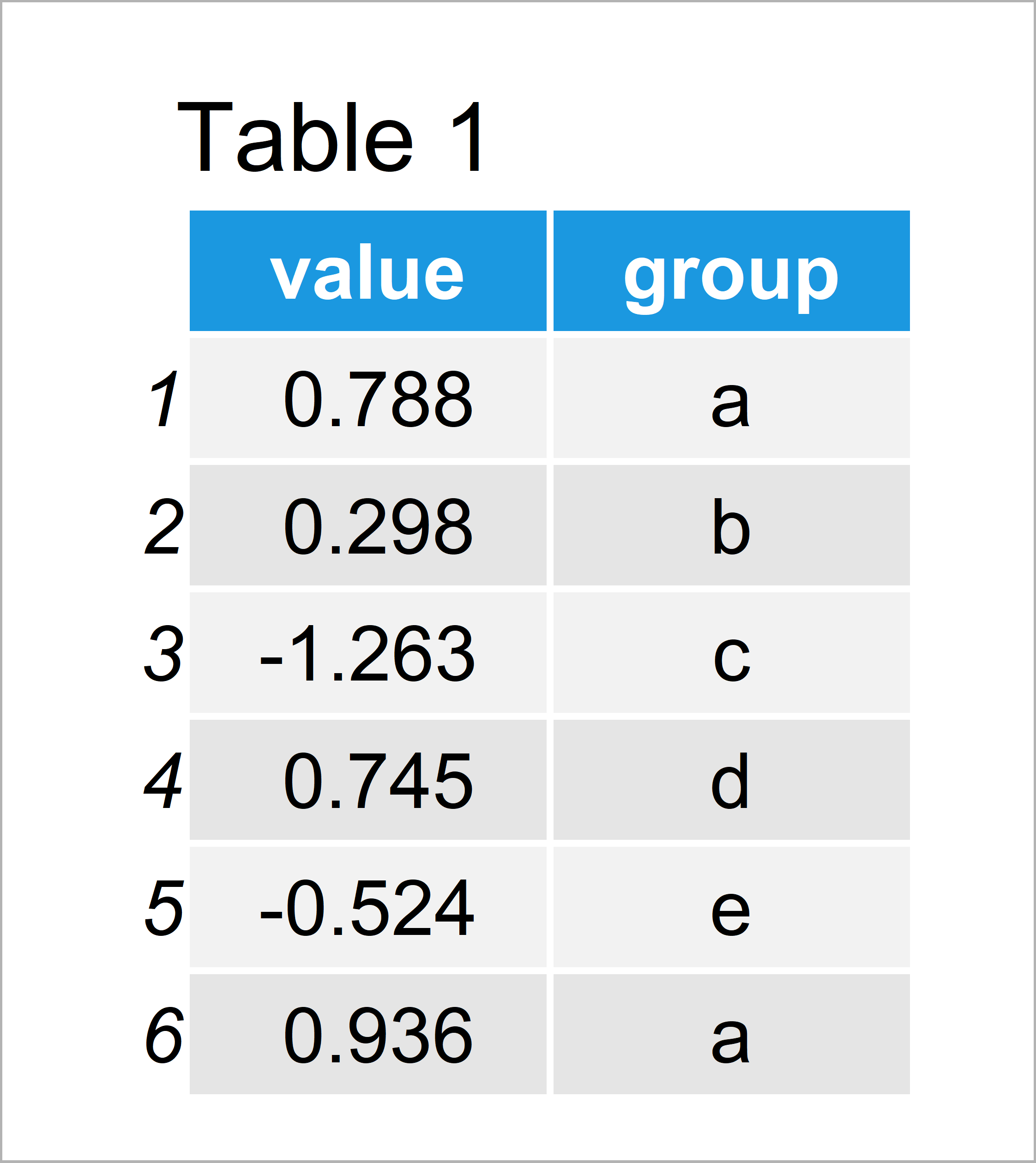# Change Color of ggplot2 Boxplot in R (3 Examples)

In this tutorial you’ll learn how to set the colors in a ggplot2 boxplot in the R programming language.

The tutorial will contain this:

Let’s dive right into the examples…

## Exemplifying Data, Packages & Basic Graph

The first step is to define some data that we can use in the examples below.

```set.seed(29384732)                                       # Create example data
data <- data.frame(value = rnorm(100),
group = letters[1:5])The previously shown table shows that our example data is constituted of 100 rows and two variables.

We also need to install and load the ggplot2 package, to be able to use the functions that are contained in the package:

```install.packages("ggplot2")                              # Install & load ggplot2 package
library("ggplot2")```

Next, we can draw our data in a boxplot without colors:

```ggplot(data, aes(x = group, y = value)) +                # Draw ggplot2 boxplot without colors
geom_boxplot()```Figure 1 shows the output of the previous R programming code: A ggplot2 box-and-whisker graph without any colors.

## Example 1: Change Border Colors of ggplot2 Boxplot

This example illustrates how to add colors to the borders and lines of the boxes of our ggplot2 boxplot.

For this, we have to specify the col argument within the aes function to be equal to our groups:

```ggplot(data, aes(x = group, y = value, col = group)) +   # Change color of borders
geom_boxplot()```By executing the previous syntax, we have created Figure 2, i.e. a boxplot with different colors for the borders and lines of each box.

## Example 2: Change Filling Colors of ggplot2 Boxplot

In this example, I’ll explain how to modify the filling colors of the boxes.

The only thing we need to change compared to Example 1 is that we have to use the fill argument instead of the col argument:

```ggplot(data, aes(x = group, y = value, fill = group)) +  # Change filling color
geom_boxplot()```By running the previous R code, we have managed to create Figure 3, i.e. a boxplot with different filling colors for each box.

Note that we could specify both the col and fill arguments at the same time to color the borders and fillings of the boxes simultaneously.

## Example 3: Manually Specify Filling Colors of ggplot2 Boxplot

So far, we have only used the default color palette of the ggplot2 package. Example 3 explains how to add user-defined colors to our plot.

For this task, we have to use the scale_fill_manual function as shown below:

```ggplot(data, aes(x = group, y = value, fill = group)) +  # Manually specified filling color
geom_boxplot() +
scale_fill_manual(breaks = data\$group,
values = c("#1b98e0", "#353436", "yellow", "red", "green"))```As shown in Figure 4, we have plotted a ggplot2 boxplot with manually defined color palette.

## Video & Further Resources

Have a look at the following video which I have published on my YouTube channel. I illustrate the R programming code of this tutorial in the video.

Please accept YouTube cookies to play this video. By accepting you will be accessing content from YouTube, a service provided by an external third party.If you accept this notice, your choice will be saved and the page will refresh.

Besides that, you could have a look at the other tutorials of this website. You can find some tutorials on topics such as graphics in R, colors, lines, and plot legends below.